Units in Electricity and Magnetism

The tables below list the systems of electrical and magnetic units. They only include units of interest in the field of Radio.
The older systems were the CGS and Gaussian systems.The Gaussian system being based on a mix of Electrostatic units (ESU) and Electromagnetic units (EMU).

The current standard is the International System of Units (SI) and is sometimes referred to as rationalised MKS units.
Conversions from one system to others are given in two ways. Firstly numerically by multiplying factors. Note that c stands for  the velocity of light in space and its value is exactly 299792458 metre/second exactly (by definition of the metre).

The other method of conversion allows you to change formulas given in old books into the modern SI form. This will be particularly useful to you if, like me, you have books by Terman, Scroggie, etc. or the Admiralty Handbook of Wireless Telegraphy. There were lots of useful formulas in these old books, which can now be given new life.

I have tried to make the tables as complete and accurate as possible and have checked lots of different sources. Nevertheless there may be errors and I will be grateful for any corrections or additions. Please email me at the address given on the home page.

SI  Units

 Quantity Symbol Unit & (Abbr.) Dimensions ESU EMU Mass m kilogram (kg) M 1000 1000 Length l metre (m) L 100 100 Time t second (s) T 1 1 Power P watt (W) ML2T-3 107 107 Electric Current I ampere (A) I=M½L1½T-2 10c 0.1 Charge Q coulomb (C) TI 10c 0.1 Electric Potential V volt (V) ML2T-3I-1 106/c 108 Resistance R ohm (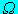) ML2T-3I-2 105/c2 109 Conductance G siemens (S) M-1L-2T3I2 10-5c2 10-9 Inductance H henry (H) ML2T-2I-2 105/c2 109 Capacitance C farad (F) M-1L-2T4I2 10-5c2 10-9 Electric Field Strength E volt/metre (V m-1) MLT-3I-1 104/c 106 Electric Charge Density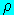coulomb/cu.m. (C m-3) L-3TI c/105 10-7 Electric displacement Electric flux density D coulomb/sq.m. (C m-2) L-2TI 4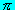10-3c 410-5 Magnetic Potentialampere (A) I 410c 410-1 Magnetic Field Strength H ampere/metre (A m-1) L-1I 4c/10 410-3 Magnetic flux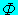weber (Wb) ML2T-2I-1 106/c 108 Magnetic Induction Magnetic Flux Density B tesla (T) MT-2I-1 100/c 104 Magnetic susceptibility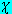e none none 10-4c-2/(4) 1/(4) Magnetic Moment m ampere metre2 (A m2) L2I 105c 103 Magnetisation (Magnetic moment/unit volume) M ampere/metre (A m-1) L-1I 10-1c 10-3 Magnetic Polarisation J tesla (T) MT-2I-1 102/(4c) 104/(4) Magnetic Pole Strength P ampere metre (A m) LI 103c 10 Magneto Motive Force Fm ampere (A) I 410c 410-1 Magnetic Reluctance S ampere/weber(A Wb-1) I2M-1L-2T2 410-9 410-9 Permittivity of spacefarad/metre (F m-1) M-1L-3T4I2 - - Permeability of space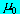henry/metre (H m-1) MLT-2I-2 - -

Notes:
c (the speed of light) = 299792458 metre/second exactly (by definition of the metre)= 1/(410-7c2) = 8.85418781762039 x 10-12 farad/metre (approx)= 410-7 = 1.25663706143592 x 10-6 henry/metre (approx)

CGS Units

 Quantity Symbol Dimensions Dimensions ESU EMU Mass m M M gram gram Length l L L centimetre centimetre Time t T T second second Power P ML2T-3 ML2T-3 erg/second erg/second Electric Current I I M½L1½T-2 statamp biot Charge Q TI M½L1½T-1 franklin abcoulomb Electric Potential V ML2T-3I-1 M½L½T-1 statvolt abvolt (lines/second) Resistance R ML2T-3I-2 L-1T statohm abohm Conductance G M-1L-2T3I2 LT-1 statsiemens absiemens Inductance L ML2T-2I-2 L-1T2 stathenry abhenry Capacitance C M-1L-2T4I2 L cm abfarad Electric field strength E MLT-3I-1 M½L-½T-1 statvolt/cm abvolt/cm Electric displacement D L-2TI M½L1½T-1 statcoulomb cm-2 abcoulomb cm-2 Dielectric constant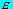M-1L-3T4I2 none none none Magnetic pole P LI M½L2½T-2 unit pole dyne cm-1 = 4lines of force Magnetic PotentialI M½L1½T-2 gilbert Magnetic field strength H L-1I M½L½T-2 oersted Magnetic fluxML2T-2I-1 M½L½ maxwell = 1 line Magnetic Induction (Magnetic flux density) B MT-2I-1 M½L-1½ gauss = 1 line cm-2 Magnetic permeability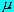MLT-2I-2 L-2T2 darcy Magnetic susceptibility(or k) M½L1½T-2I-1 none none none Intensity of Magnetisation M (or I) = J L-1I M½L½T-2 pole cm-2 Magnetic Moment m L2I M½L3½T-2 pole cm Magneto Motive force G I M½L1½T-2 gilbert Magnetic Reluctance S L-2M-1T2I2 LT-2 gilbert/biot

Notes:
In the first column of Dimensions, I is used as a basic unit.
In the second it is expressed in terms of Length, Mass and Time.

Gaussian Units

The Gaussian system uses a mix of Electrostatic and Electromagnetic units
There are two common forms, Gaussian and Modified Gaussian, which defines electric current in terms of magnetic units

 Quantity Symbol Gaussian Modified Gaussian Electric Current I ESU franklin EMU biot Charge Q ESU statcoulomb ESU statcoulomb Electric Potential V ESU statvolt ESU statvolt Resistance R ESU statohm ESU statohm Inductance L EMU abhenry cm Capacitance C cm cm Electric field strength E ESU statvolt/cm ESU statvolt/cm Electric displacement D ESU statcoulomb/cm2 ESU statcoulomb/cm2 Dielectric constantnone none Magnetic pole P EMU unit pole EMU unit pole Magnetic PotentialEMU gilbert EMU gilbert Magnetic field strength H EMU oersted EMU oersted Magnetic fluxEMU maxwell = 1 line EMU maxwell = 1 line Magnetic Induction (Magnetic flux density) B EMU gauss EMU gauss Magnetic permeabilityEMU darcy EMU darcy Magnetic susceptibilitynone none Intensity of Magnetisation M EMU pole cm-2 EMU pole cm-2 Magnetic Moment m EMU pole cm EMU pole cm Magneto Motive force G EMU gilbert EMU gilbert Magnetic Reluctance S EMU EMU

Conversion of Gaussian formulae into SI

To convert a formula from the Gauss form into the SI form, replace the elements on both sides of the equation using the table below. Mass, Length, Time and others not listed below are not changed.

 Quantity Gaussian SI Electric Current I I /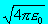Electric Current in EMU I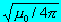I Charge Q Q /Electric Potential, PD, EMF VV Resistance R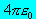R Inductance LL Capacitance C C / () Electric field strength EE Electric displacement D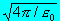D Dielectric constant/Magnetic pole PP Magnetic PotentialMagnetic field strength HH Magnetic flux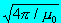Magnetic Induction (Magnetic flux density) BB Magnetic permeability/Magnetic susceptibilityor ke Intensity of Magnetisation IM Magnetic Moment mm Magneto Motive force GG Magnetic Reluctance SS

Maxwell's equations

In Gaussian units: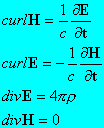On conversion to SI units these become: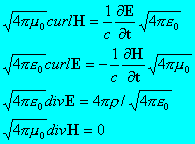On simplifying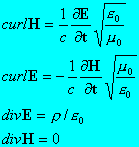Then using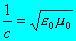we get: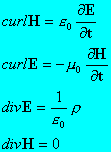Approximate ESU/EMU units in terms of SI units

 Quantity 1 ESU unit 1 EMU unit Current 334A 10 A Charge 334C 10 C Potential 300 V 10 nV Power 100 nW 100 nW Resistance 90 G1 nConductance 1 pS 1 GS Inductance 90 GH 1 nH Capacitance 1 pF 1 GF Magnetic flux 300 Wb 10 nWb Magnetic induction 3 MT 100T Magnetic field strength 3 nA/metre 80 A/metre Magnetisation 33 nA/metre 1000 A/metre Electric field strength 30000 V/metre 1V/metre Electric displacement 265 nC/metre 8000 C/metre